### Shane Blowes and Sean Connolly. 2012. Risk spread, connectivity, and optimal reserve spacing. Ecological Applications 22:311–321.

Appendix A. Quasi-extinction analysis.

Our measure of metapopulation viability, geometric mean metapopulation growth (R), is a metapopulation’s long-run expected rate of recovery from depletion. One alternative to this measure is the diffusion approximation for the probability of quasi-extinction (decline to a pre-defined threshold population) over some time horizon. This quantity is a function of the expected temporal variance in population growth rate, as well as the geometric mean growth rate. Although the analytical expressions for quasi-extinction were derived from theory for single populations undergoing stochastic density-independent growth (Lande & Orzack 1988, Dennis et al. 1991), extensive simulation studies indicate that these expressions also provide good approximations for the quasi-extinction probabilities of metapopulations (Holmes & Semmens 2004).

Quasi-extinction probability is a function of the variance in (the natural logarithm of) metapopulation growth rate, as well as its geometric mean. To estimate this variance in each of our simulations, we first estimated the natural log of the geometric growth rate for each time step after discarding the first 100 time steps:

 μi, t = log(Mi, t + 1) - log(Mi, t) (A.1)

where Mt is the metapopulation size in the ith simulation at time t. Variance in metapopulation growth for simulation i (si2) was then determined by calculating the variance of μi, t, and finally σ2 was estimated by taking the mean of si2 over all 1000 simulations.

Using a diffusion approximation, the cumulative distribution function for the time to quasi-extinction (Eq. A.2) gives the probability that some critical population size is reached at any time between t = 0 and t = T: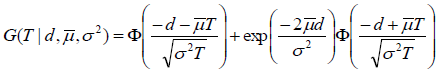(A.2)

where μ is the natural log of the geometric mean metapopulation growth rate, d is the natural logarithm of the ratio between the starting metapopulation size (M0) and the critical population size (Mc), and Φ is the standard normal cumulative distribution function with a mean of zero and variance of 1. In the figures below, we have set d = M0/Mc = 5, which corresponds to a threshold population size that is 20% of the initial population size. For the 10-year time horizon, such a decline would warrant classification as “Critically Endangered” (i.e., 80% decline over 10 years: Criterion A4 of the IUCN Red List). We explored several alternative threshold population sizes, and this appeared to have negligible effects on the qualitative results shown below (number and location of the peaks in the probability of persistence).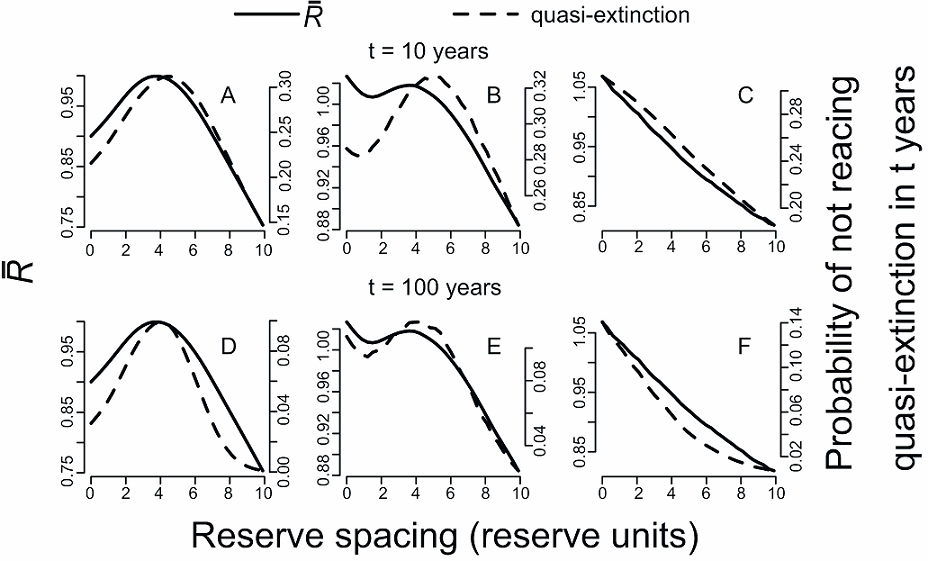FIG. A1. Quasi-extinction risk and (for comparison) geometric mean population growth rate as a function of inter-reserve distance. Parameters in all panels are equal to baseline parameters in Figure 1. Panels (A-C) show probability of the metapopulation not declining to 80% of starting its starting size in 10 years for: (A) Gaussian dispersal and Gaussian covariance, (B) Laplacian dispersal and Gaussian covariance, (C) Laplacian dispersal and exponential covariance. Panels (D-F) show the probability of the metapopulation not declining to 80% of its starting size in 100 years for: (D) Gaussian dispersal and Gaussian covariance, (E) Laplacian dispersal and Gaussian covariance, (F) Laplacian dispersal and exponential covariance.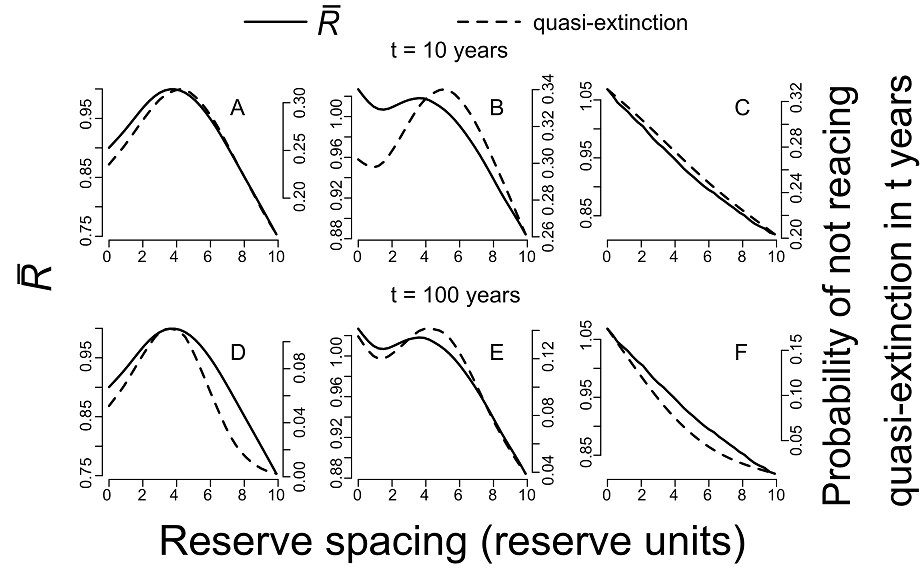FIG. A2. Effect of temporal variation in diffusion on quasi-extinction risk and (for comparison) geometric mean population growth as a function of inter-reserve distance for the three different qualitative outcomes as shown in Figure 6(A-C). In all panels: E(ln[fA]) = E(ln[fB]) = 1.9, σA2 = σB2 = 2.2, y = 0, and diffusion (a) is lognormally distributed with µa = 6,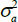= 4. Panels (A-C) show the probability of the metapopulation not declining to 80% of starting its starting size in 10 years for: (A) Gaussian dispersal and Gaussian covariance (Φ = 5); (B) Laplacian dispersal and Gaussian covariance (Φ = 5); (C) Laplacian dispersal and exponential covariance (Φ = 5√3). Panels (D-F) show the probability of the metapopulation not declining to 80% of starting its starting size in 100 years for: (D) Gaussian dispersal and Gaussian covariance (Φ = 5); (E) Laplacian dispersal and Gaussian covariance (Φ = 5); (F) Laplacian dispersal and exponential covariance (Φ = 5√3).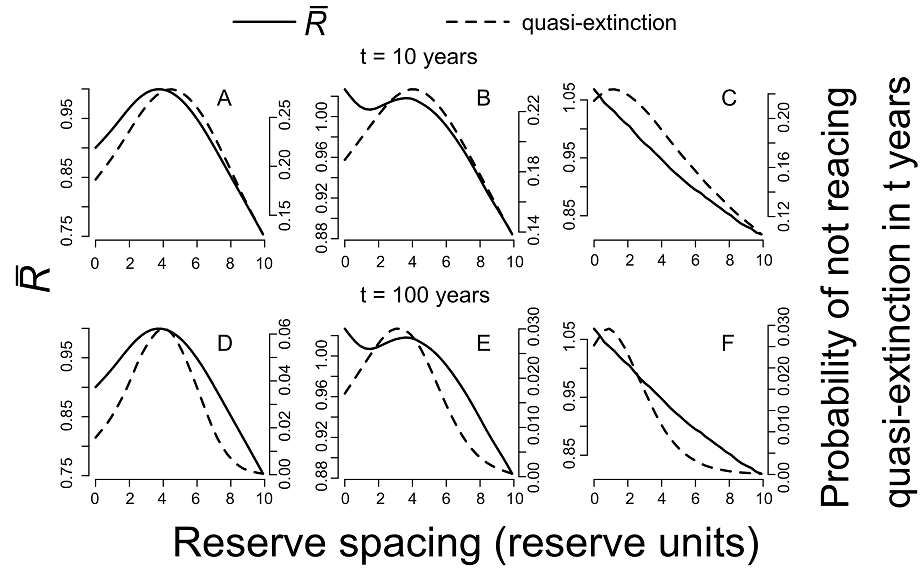FIG. A3. Effect of temporal variation in advection on quasi-extinction risk and (for comparison) geometric mean population growth as a function of inter-reserve distance for the three different qualitative outcomes as shown in Figure 6(D-F). In all panels: E(ln[fA]) = E(ln[fB]) = 1.9, σA2 = σB2 = 2.2, a = 6, and advection (y) is normally distributed with µa = 0,= 4. Panels (A-C) show the probability of the metapopulation not declining to 80% of starting its starting size in 10 years for: (A) Gaussian dispersal and Gaussian covariance (Φ = 5); (B) Laplacian dispersal and Gaussian covariance (Φ = 5); (C) Laplacian dispersal and exponential covariance (Φ = 5√3). Panels (D-F) show the probability of the metapopulation not declining to 80% of starting its starting size in 100 years for: (D) Gaussian dispersal and Gaussian covariance (Φ = 5); (E) Laplacian dispersal and Gaussian covariance (Φ = 5); (F) Laplacian dispersal and exponential covariance (Φ = 5√3).

LITERATURE CITED

Dennis, B., P. L. Munholland, and J. M. Scott. 1991. Estimation of growth and extinction parameters for endangered species. Ecol. Mono. 61:115–143.

Holmes, E. E., and B. X. Semmens. 2004. Viability analysis for endangered metapopulations: a diffusion approximation approach. Pages 565–597 in I. Hanski, and O. E. Gaggiotto, editors. Ecology, genetics, and evolution of metapopulations. Elsevier Academic Press, Burlington, MA.

Lande, R., and S. H. Orzack. 1988. Extinction dynamics of age-structured populations in a fluctuating environment. Proc. Nat. Acad. Sci. 85:7418–7421.M

Morris, W. F., and D. F. Doak. 2002. Quantitative conservation biology: theory and practice of population viability analysis. Sinauer Associates Sunderland, MA.

[Back to A022-019]